Egg Curves and Ovals
 What are the Oval and the Egg Curve? Ellipses and its changings  More Curves as Loci of Points Chains of  Eggs Egg Curves with Arcs Section through Rotation Shapes More Curves References Egg Curves on the Internet .
 To the Main Page  "Mathematische Basteleien"

What are an Oval and an Egg Curve?
There is no clear definion. Mostly you define:
 ...... An oval is a closed plane line, which is like an ellipse or like the shape of the egg of a hen. An egg curve only is the border line of a hen egg.  The hen egg is smaller at one end and has only one symmetry axis.

The oval and the egg shaped curve are convex curves, differentiate twice and has a positive curvature.

 ...... You distinguish between the oval, the ovoid and the oval shape in the same way as between the circle, the figure of the circle and the sphere.

Ellipses and its changings top
Ellipse
All points P, for which the distances of two fixed points or foci F1 and F2 have a constant sum, form an ellipse. The ellipse  in the centre position has the following cartesian equation.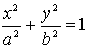The parameters a and b are called lengths of axis.
The ellipse is the formula of a relation.
......

The ellipse on the left has the equationThe constant sum is 2a=6.... You can add two halves of different ellipses to form a chicken egg.

A Gardener's Construction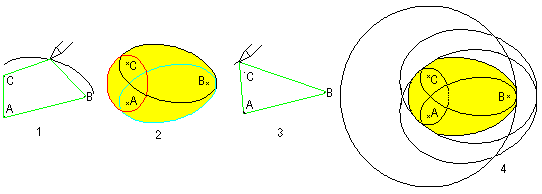You can draw an egg curve, if you wrap a rope (green) around an isosceles triangle and draw with taut rope a closed line (1). The rope must be a little bit longer than the circumference of the triangle. Elllipse arcs develop, which together form an egg shaped curve (2).

The three main ellipse are totally drawn in a computer simulation (2,black, red, blue, book 9). You are exacter, if you draw three more ellipses in the sector of the vertical angles of the triangle angles to the sides AB, AC und BC (3,4).

Another Gardener's Construction
 ...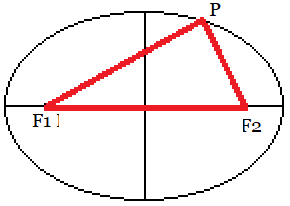... If a gardener wants to create an oval bed, he proceeds as follows: He drives two stakes into the ground and wraps a ribbon around them as in the drawing. He sticks a stick, which serves as a pencil, into the ground and guides it around the stakes. He makes sure that the string is taut. This creates an ellipse.

 ...... If the stake F1 is replaced by a stake with a large diameter, the result is a egg shaped curve. I confirmed this in a small experiment with a medicine cup, needle, wool thread, ball pen on cardboard.

Gerhard Rörik has studied this kind of curves.
 ...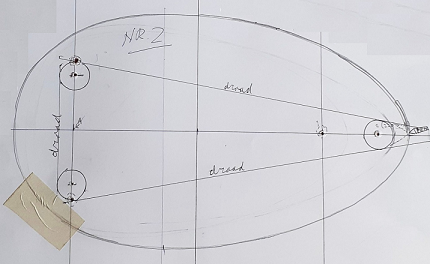... He examined curves around two or three "logs" of different diameters.
I thank him for pointing out this method of creating a egg shaped line.
2022 June

Super Ellipse
...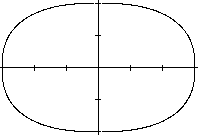... If you take the exponent 2.5 instead of  2 in the equation (x/a)²+(y/b)²=1, you get the equation of a super ellipse:The modulus | | makes sure that the roots are defined.
In the drawing there is a=3 and b=2.
The Danish author and scientist Piet Hein (1905-1996) dealt with the super ellipse in great detail (book 4). In particular that the shape made by rotation around the x-axis can stand on the top, if it is made from wood. You don't have to use power in contrast to the Columbus' egg.
The super ellipse belongs to the Lamé curves. They have the equations...... In the drawing there is a=3, b=2 and you substitute n with 1(parallelogram, blue), 1.5(green), 2(Ellipse, bright red), 2.5 (super ellipse, red), and 3 (black).

From the Oval to the Egg Shape
You can develop the shape of a hen egg, if you change the equation of a oval a little. You multiply y or y² by a suitable term t(x), so that y becomes smaller on the right side of the y-axis and larger on the left side. y(x=0) must not be changed.
The equation of the ellipse e.g. x²/9+y²/4=1 change to x²/9+y²/4*t(x)=1. Here you multiply y² with t(x).
Three examples:To the red egg shaped curve:
The ellipse is black. The egg curve is red. It lies under the ellipse on the right side of the y-axis. The term there is larger than 1. The number 4 (=b²) becomes smaller by multiplication of y²/4. So the curves belongs to ellipses with smaller minor axes. It is under the black ellipse.
Corresponding you explain, why the red curve lies above the black ellipse on the left of the y-axis. (You multiply with a number smaller than 1...)

To the blue and green egg curves:
They have about the same shape, though the equations are different at first glance.
But:
t2(x)=1/(1-0,2x) can be written as geometric series.
Generally there ist 1/(1-q) = 1+q+q²+..., here is 1/(1-0,2x) = 1+0,2x+0,04x²+...

t3(x)=exp(0.2x) kann be developped as Taylor's series
Generally there is f(x) = f(0)+x*f'(0)+x²*f''(0)+..., here is exp(0.2x) = 1+0,2x+0,02x²+...

To compare t1(x)=1+0,2*x+0*x².

The three terms  t1, t2 und t3 differ in the series not until in the square term.

 Further there is t1

From the Egg to the Triangle
 ...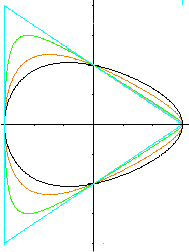... If you substitute the term t(x)=(1+kx)/(1-kx) in the equation  x²/9+y²/4*t(x)=1, you get the curves on the left for different numbers k.  black: k=0,1       red: k=0,2      green: k=0,3       blue k=1/3. The black egg becomes a blue triangle.  The black egg is the same as those of  t1(x), t2(x) oder t3(x) above, because the geometric series (1+0,1x)/(1-0,1x)=1+0.2x+0.02x²+... correspondend to the first terms. You get a triangle for k=1/3. a=3 is the major axis.  Proof: The equations x²/a²+y²/b²*(1+x/a)/(1-x/a)=1 and (x/a+y/a-1)(x/a-y/b-1)(x/a+1)=0 are equivalent. If you simplify both terms, you get  -b²x³+ab²x²+a²b²x+a²xy²+a³y²-a³b²=0.
The 3 lines of the triangles are described by the 3 factors in (x/a+y/b-1)(x/a-y/b-1)(x/a+1)=0

Letter
 ...... Don M. Jacobs, M.D., from Daly City, USA developped a nice egg shape by changing the circle equation x²+y²=1 a little: x² + [1.4^x*1.6y]² = 1. The egg equation is an exponential equation of the type t3. This shows this conversion: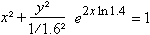.

Inversion of an Ellipse in a Circle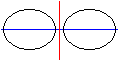... If you reflect an ellipse in a straight line, you get an ellipse again (on the left). If you reflect an ellipse in a circle, you get an egg curve (on the right).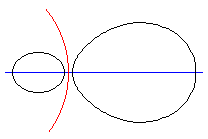An inversion is the function of the Argand plane one-one by reciprocal radii or a reflection in a circle with the radius R. The centre of the reflection is the origin (0|0). The equation of the function is z'=R²/z.

More Curves as Loci of Points  top
Cassini Ovals
All points P, for which the distances of two fixed points or foci F1 and F2 have a constant product, form a Cassini oval. The Cassini oval has the following Cartesian equation in the centre position (x²+y²)² - 2e² (x²-y²) - (a²)² + (e²)²=0.
2e is the distance of both fixed points, a² is the constant product.
 ...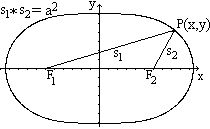... The curve on the left has the equation  (x²+y²)² - 72(x²-y²) - 2800 = 0. There is e=6, a=8.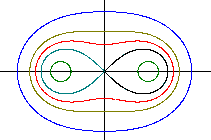This drawing originated from fixing e=6 and substituting a =10 (blue), 8.5 (grey), 7 ( red), 6 (black) und 4 (green) in the formula.
Generally said:
If a>[e multiplied by the square root of 2]  there is a egg figure.
If a=[e multiplied by the square root of 2]  there also is an egg figure, but the curvation is 0 on the vertical axis.
If e<a<[e multiplied by the square root of 2] there is a figure cut into the middle.
If a=e there is a lemniskate.
If a<e there are two ovals.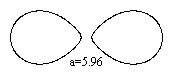The ovals inside with a

Cartesian Ovals
All points, for which the simple and the double distances of two fixed points or foci F1 and F2 have a constant sum, form a Cartesian oval. The Cartesian oval has the following cartesian equation.
4a²m²((c-x)²+y²)-(a²+m²c²-2cm²x+(m²-1)(x²+y²))²=0
c is the distance of the fixed points and m=2 ("double distance"). The origin of the coordinate system is the left fixed point.
This long equation is derived with the formulation s1+2*s2=a and by using Pythagoras' formula twice.
 ...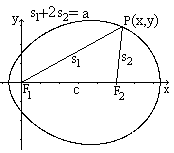... The distance of the fixed points is c=5 and the sum a=12. The equation is now 2304((5-x)²+y²) - (3x²+3y²-40x+44)²=0.
 ......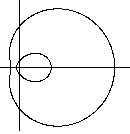........ The graph from above is incomplete. Surprisingly the equation 2304((5-x)²+y²)-(3x²+3y²-40x+44)²=0 produces another curve outside the egg curve.
 ........... If you substitute m=2 with m=2.2, you produce another egg shape. You keep c=5 and a=12.
These egg curves go back to Renatus Cartesius alias René Descartes (1596-1650), therefore the name.

Curves by Loops
 Szegö Curve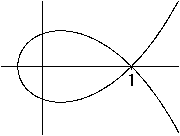x²+y²=e2x-2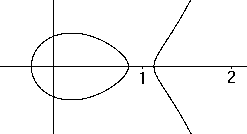x²+y²+0,02=e2x-2 Folium of Descartes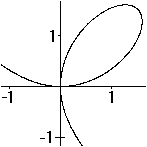x³+y³=3xy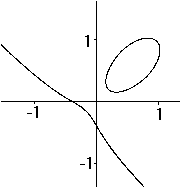x³+y³+0,06=3xy(x²+y²)³-4x²y²(x²+y²)³+0,001-4x²y² More egg curves this way: >Trisextrix of MacLaurin y²(1+x)+0,01=x²(3-x) >Lemniskate of Bernoulli (x²+y²)²-(x²-y²)+0,01=0 >Conchoid of de Sluze 0,5(x+0,5)(x²+y²)-x²+0,02=0 ...
(Torsten Sillke's idea)
Drawing by Fritz Hügelschäffer

Transfer the well known drawing of an ellipse with the help of two concentric circles (on the left) to a two-circle-figure.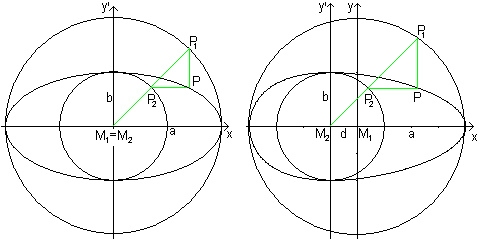Draw in the order M1, M2 , P1, P2 , and P.
a and b are the radii of the circles, d is the distance of its centres.
The parameters a, b, c are suitable to describe the egg shape. 2a is its length, 2b its width and d shows the broadest position.

The equation of the egg shaped curve is an equation of third degree:
x²/a² + y²/b²[1 + (2dx+d²)/a²] = 1
b²x²+a²y²+2dxy²+d²y²-a²b²=0

The drawn egg shaped curve has the parameters a=4, b=2 und d=1. The equation is 4x²+16y²+2xy²+y²-64=0.

Second example:In this example there is a=4, b=3 and d=1.  The equation is 9x²+16y²+2xy²+y²-144=0.
Origin: (11), page 67/68

Granville's Egg Curve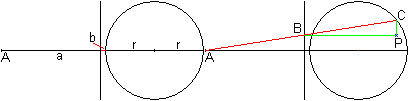>There is given a line, which starts at point A and lies horizontally. Then there is a vertical line in the distance of a and a circle with the radius r being symmetric to the horizontal line in the distance of a+b (drawing on the left).
>If you draw a line (red) starting at point A, it cuts the vertical line at B and the circle at C. If you draw then a vertical line through C and a horizontal line through B (green), they meet at P.
>If the point C moves along the circle, then the points P lies on a egg shaped curve (animation on the right).
See more: (13),  Jan Wassenaar (Granville's egg, URL below), Torsten Sillke (Granville's egg, URL below)

Mechanical Egg Curve Construction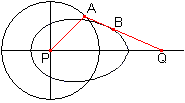Let P be a fixed point and A a point, which moves on a circle around P with the radius r=PA.  Link the bar a=QA at A . Its free end Q moves on a horizontal through P forth and back. The point B on the line AQ with BQ=b describes an egg shaped curve.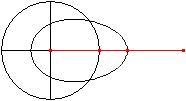See more: (12), www.museo.unimo.it/theatrum/macchine/, Jan Wassenaar (quartic egg curve, URL unten)

Chains of  Eggs top
A Double Egg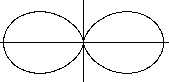... The polar form r(t)=cos²t produces a double egg  (Münger 1894).  A second equation is r(t)=exp(cos(2t))*cos²(t) (Hortsch 1990).

Another Double EggThe Equation x4+2x²y²+4y4-x³-6x²-xy²=0 produces a double egg.
There is a wide field of experimenting.

Chains
You can form and combine sinus curves in such a way, that you get a chain of eggs.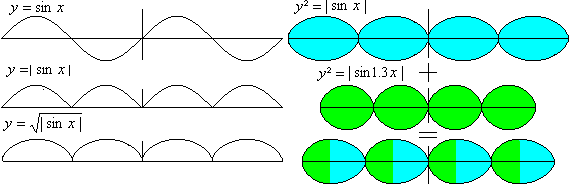Also polyominals can produce chains (see Torsten Sillke, URL below).

The equation y² = abs[sin(x)+0.1sin(2x)] describes a sinus chain more elegant:(Torsten Sillke)

Egg Curves with Arcs  top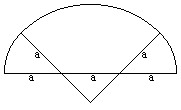...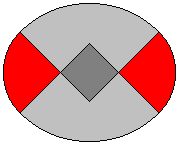... Two small (red) and two big (grey) quarter circles, which have a square in common,  form an oval. (The angles of the sectors don't have to be 90°.)
 ...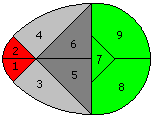... A semi-circle (green), a quarter circle (red) and two eighth circles (grey), which have a triangle in common, form a second figure. If you cut the egg in nine pieces, you get the tangram puzzle "The Magic Egg" or "The Egg of Columbus".

 ...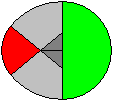... You can generalize the figure: Take a smaller dark grey triangle.

 ...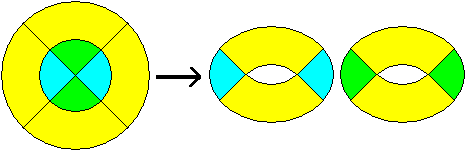Divided and reassembled again

 ...... Divided and reassembled again. (14), Seite 122..

An egg inside a pentagon - almost
 ...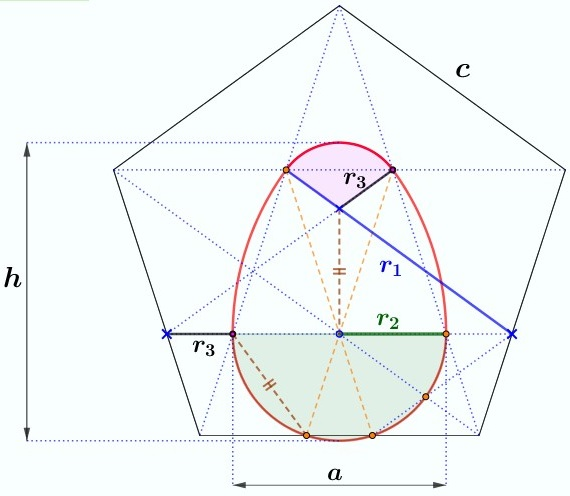... r1 = c r2 = c(2-phi) r3 = c(2phi-3) h =(c/phi)[1+sqrt(7-4phi)] a = 2c(2-phi)  phi = (1/2)[1+sqrt(5)] ............................................................................................
Fernand Marsal sent me this figure in June 2022, and the formulas also come from him.

Section through Rotation Shapes  top
If you make a sloping section through a cone or a cylinder you often get an ellipse as a section line. If you choose an hyperbolic funnel, you get egg curves in the form of a hen egg. Hyperbolic funnels are figures, which develop from rotation of an hyperbola around the symmetry axis.... There is the hyperbolic funnel to f(x) =1/x². The y-axis is perpendicular to the x-z-plane in direction to the back.  The straight line shows the section plane perpendicular to the x-z-plane.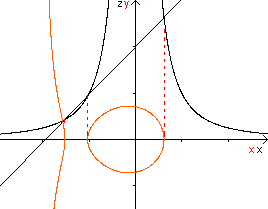... The given plane intersects the hyperbolic funnel with three points in the x-z-plane. If you project the section lines in the x-y-plane you get the red curves.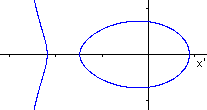... You get an egg curve in the section plane.

Formulas:If you make a sloping section through other figures, you get more egg curves.

More Curves   top
Equations of 3rd and 4th Degree
 ...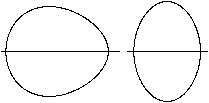... Equations with the form y²=p(x-a)(x-b)(x-c)... produce egg curves.  There are two examples on the left: 2y²=(x-1)(x-2)(x-3)  and  y²=(x-1)(x-2)(x-3)(x-4)

The Folium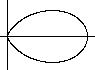... The polar form r(t)=cos³t produces the folium or wrong Kepler egg.

A Crooked Egg
"Jedes legt noch schnell ein Ei und dann kommt der Tod herbei."
"Each still lays a final egg, then comes Death and out they peg." (Reclam)
 ...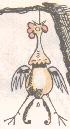... The polar equation r(t)=sin³t+cos³t produces a crooked egg ;-).Upright Egg
 ...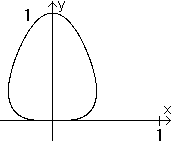Torsten Sillke's egg of Columbus  y^4+10y^2x^2+5x^4 = y

New eggs
These egg curves were discovered by Florian Blaschke (E-Mail from 02.07.2016).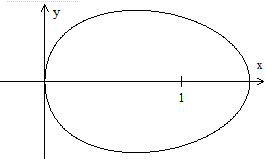x^1.5-1.5^0.5x+y^2 = 0x^1.5-a^0.5x+y^2 = 0 and a=1, 2, 3

New egg
 ...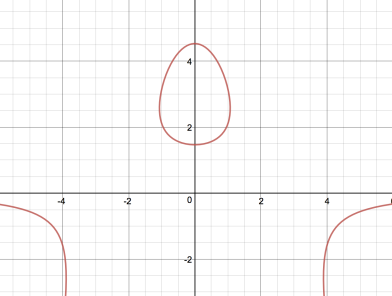This egg curve was discovered by Florian Blaschke (E-Mail from 23.09.2018)...... 2y(x^2)+e*(y-3)^2=6.4 e is the Euler number.

New egg
 ...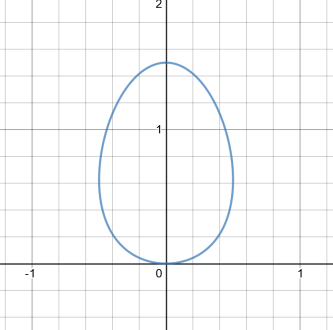Adrian Skovgaard discovered this web page and sent me another egg.  x^2 = 3*sqrt(2y+1)-2y-3 (Email sent on April 27th 2020)

New egg
 ...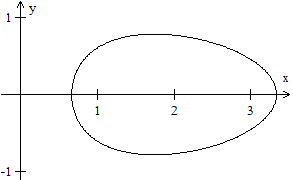Jean-Claude Babois found an equation in which "phi" occurs from the golden ratio.  y^2+(1/phi)*[sqr( x)-1/phi]^2=(1/10)*(1/phi). (Email zugesandt am 20.12.2022)

New egg
 ...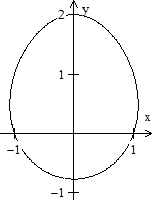... Arthur Bouma sent me, as he wrote, the by far simplest equation of an egg shaped curve.                                               x^2+y^2 = 2^y (Email sent to me on 17.08.2023)

References  top

English:

(1) Lockwood, E. H.: A Book of Curves.
Cambridge, England: Cambridge University Press, p. 157, 1967.

(2) Martin Gardner: The Last Recreations, Hydras, Eggs, and Other Math.Mystifications, Springer,  New York  1997

German:

(3) Sz.-Nagy, Gyula: Tschirnhaussche Eiflaechen und Eikurven. Acta Math. Acad. Sci. Hung. 1, 36-45 (1950). Zbl 040.38402

(4) Ulrich/Hoffman: Differential- und Integralrechnung zum Selbstunterricht, Hollfeld 1975

(5) Martin Gardner: Mathematischer Karneval, Frankfurt/M, Berlin 1977

(6) Gellert...: Kleine Enzyklopädie - Mathematik, Leipzig 1986

(7) Wolfgang Hortsch, Alte und neue Eiformeln in der Geschichte der Mathematik, Muenchen, Selbstverlag 1990, 30S

(8) Gebel und Seifert, Das Ei einmal anders betrachtet, (eine Schülerarbeit) Junge Wissenschaft 7 (1992)

(9) Hans Schupp, Heinz Dabrock:   Höhere Kurven,  BI Wissenschaftsverlag 1995

(10) Gardner, Martin: Geometrie mit Taxis, die Koepfe der Hydra und andere mathematische Spielereien. Basel: Birkhaeuser (1997), Deutsche Ausgabe von (2)

(11) Elemente der Mathematik 3 (1948)

(12) Karl Mocnik: Ellipse, Ei-Kurve und Apollonius-Kreis, Praxis der Mathematik. (1998) v. 40(4) p. 165-167

(13)W. A. Granville: Elements of the differential and integral calculus, Boston, (1929)

(14) Heinz Haber (Hrsg.): Mathematisches Kabinett, München 1983 [ISBN 3-423-10121-0]

Egg Curves on the Internet  top

Deutsch

Michael Hinterseher
Eilinien(mit Klotoiden)

Projekt der Universität Würzburg
Mathematik rund ums Ei

Wikipedia
Oval (Geometrie), Ei-Kurve, Ellipse, Superellipse, Cassinische Kurve, Ei des Kolumbus

Englisch

André Heck
A potpourri of mathematical egg curves

CARLOS CALVIMONTES ROJAS
GEOMETRY OF THE PARABOLA ACCORDING TO THE GOLDEN NUMBER

Chickscope project at the Beckman Institute
Eggmath

Eric W. Weisstein (MathWorld)
Oval, Cartesian Ovals, Cassini Ovals, Ellipse, Cundy and Rollett's EggMosss EggLemon, Superellipse

Jan Wassenaar
2dcurves

Paul L. Rosin
On the Construction of Ovals

Richard Parris (Freeware-Programme)
winplot

The MacTutor History of Mathematics archive  (Created by John J O'Connor and Edmund F Robertson)
Cassinian OvalFolium, Cartesian Oval

Torsten Sillke
Egg shaped curves
 Granville's egg - quartic [Granville 1929]  Cubic curves as perturbated ellipse Mechanical egg curve construction by a two bar linkage - a quartic  Polynomials making chains of eggs Newton's cubic: Elliptic curve  Apollonian cubic Transforming the ellipse Limacon Graphics Gallery Toric sections - hippopede of Proclus: analyzed by Perseus  The Family   r = cos^p(phi) or  [Münger Eggs]  Multifocal Curves - Tschirnhaussche Eikurven  Pivot transform construction of Path-curves Bezier Curve References

Zvonimir Durcevic
CONIC SECTIONS AND THEIR SPECIAL CASES

Französisch

Robert FERRÉOL (mathcurve)
OVOÏDE, OVALE DE DESCARTES, ELLIPSE, FOLIUM SIMPLE, OEUF DOUBLE, Oeuf d'Ehrhart,
UF DE GRANVILLECOURBE DE ROSILLOOVOÏDE

Serge MEHL
Ovale, Ovales de Cassini

Holländisch

NN (published in: Pythagoras, wiskundetijdschrift voor jongeren, december 2000)
Een eitje, zo'n eitje

Usbekistanisch

u cassini.html

Dänisch

Erik Vestergaard
Ellipser og æg, Piet Heins Superellipse

Tschechisch

Jirka Landa
Rovnice vají?ka - jednoduchá jako Kolumbovo vejceVelikonocní speciál (Video)

Japanisch
A.Gärtl, Willi Jeschke, Torsten Sillke, Gail from Oregon Coast - thanks.

Feedback: Email address on my main page